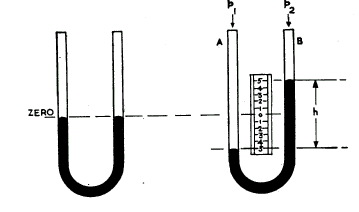# Equal Column Liquid Manometer

This is one of the oldest methods of measuring differential pressure. In its most basic configuration it consists of two identical vertical tubes partly filled with a liquid. These tubes are connected together at the bottom. Pressure is measured by determining the change in height between the two tubes and is scaled according to the density of the liquid. Thus different fluids will give a change in the pressure range.

Typical fluids used are water and mercury.

The method is very accurate however is limited to lower ranges of pressure. It is rarely used today, however it used to be used quite extensively as a calibration standard for low pressure instruments and control valves. This role has now been taken over by highly accurate calibration tools. The following diagram depicts an equal column liquid manometer. It shows a glass tube bent into a "U" shape. This tube is partly filled with the fluid and a pressure scale is located between both tubes.# The Principle of an equal column "U" tube manometer

The liquid column produces a pressure which is proportional to the height of the liquid in the leg. The pressure developed is equal to hr g (h=height/r =density/g=the acceleration due to gravity. The instrument measures the difference in pressures exerted on the liquid in each of the columns.

With higher pressure p1 being applied to column A and lower pressure p2 to B the liquid in column A is forced down until the pressure p1 is equalised by the pressure in column B. thus the difference in pressures is determined by the height of the liquid h. The scale has been calibrated as a result of the selection of the fluid used, as different fluids at different densities will give a different change in height for the same pressure applied.

That is

 p1 - p2 = h r g

Units are important, if for instance:

• pand p2 are in pascal r must be in gram/cm3 and h in meters